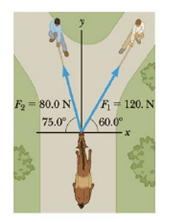Chapter 1, Problem 70P

Chapter
Section
Textbook Problem

The helicopter view in Figure P1.70 shows two people pulling on a stubborn mule. Find (a) the single force that is equivalent to the two forces shown and (b) the force a third person would have to exert on the mule to make the net force equal to zero. The forces are measured in units of newtons (N).Figure P1.70

(a)

To determine
The equivalent force.

Explanation

Given Info: The first force (F1) is having magnitude 120N and directed at an angle 60° .

Explanation:

The second force (F2) is having magnitude 80N and directed at an angle 75° .

The x component of the equivalent force is,

Fx=[(120N)cos(60)][(80N)cos(75)]=39.3N

The y component of the equivalent force is,

Fy=[(120N)sin(60)]+[(80N)sin(75)]=181N

Formula to calculate the equivalent force is,

F=Fx2+Fy2

• F is f the equivalent force
• FxandFy are x and y component of the equivalent force,

Substitute 39.3N for Fx , 181N for Fy to find F

(b)

To determine
The third force which make the net force equal to zero.

Still sussing out bartleby?

Check out a sample textbook solution.

See a sample solution

The Solution to Your Study Problems

Bartleby provides explanations to thousands of textbook problems written by our experts, many with advanced degrees!

Get Started

Find more solutions based on key concepts